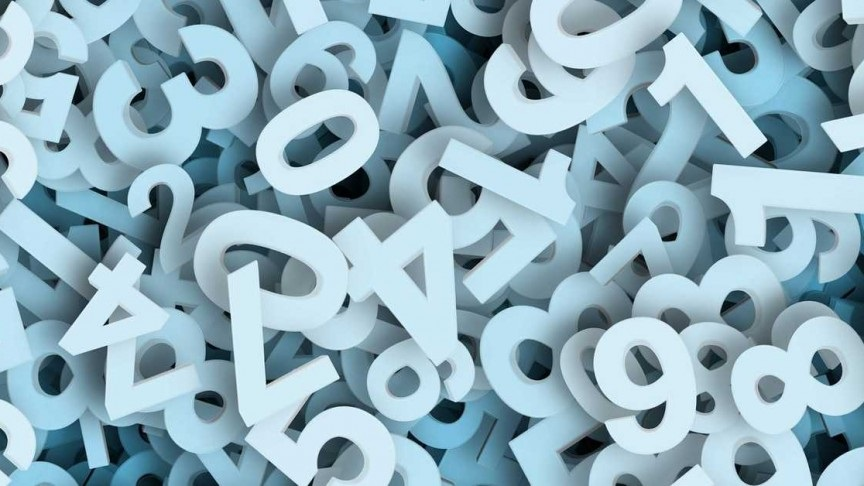Tue. Jul 27th, 2021

# Incredible Importance and Applications of NumbersNumbers play a vital role in all our lives. If we talk about numbers, the first thing that comes to our mind is “Maths”. We can say that Maths is a science with many numbers and logic. Many students find difficulty in learning mathematics, but some students really enjoy it. The numbers are important in everyday life. We use numbers to add, subtract, multiply or divide the expenses, time on the clock, date of birth, and so on.

Children of all age groups should have to learn different types of numbers in Maths. It helps them to improve their logical ability and helps them to think in an abstract way. The different types of numbers are directly or indirectly connected to everyone’s life. Kids have to learn the basic counting numbers, such as whole numbers, roman numbers 1 to 100, real numbers, natural numbers to develop their problem-solving skills. The knowledge of early number sense is one of the most important things for every child, as it helps them to understand the detailed concept of mathematics. Many important numbers in Maths are employed in the creation of new technological products. Now, let us discuss the few important numbers and its applications below:

## Pi value

The most important constant in Maths is “Pi”. It is denoted by the symbol “π”. As we know “Pi” is defined as the ratio of the circumference of the circle to its diameter. The value of Pi is approximately equal to 3.1415926563… In many mathematical problems, we use the Pi value as 3.14, which is rounded to two decimal places. Pi is considered as an irrational number, and the fractional representation of the Pi value is 22/7. In real life, Pi value is employed in different areas like trigonometry, science and nature to calculate the river bends, sound waves, light waves, and so on. Some examples of Pi value in daily uses are:

• Clock designers use Pi value while designing the pendulum for the clock.
• In Statistics, Pi value is employed to track the population dynamics.
• In electronics, electrical engineers use the value of Pi to solve the problems on electrical applications.
• In mathematics, Pi value helps to calculate the area of different geometrical figures, such as circle, sphere and so on.

## Euler’s Constant

Euler’s constant or Euler’s number has many applications in higher-level mathematics, such as calculus, statistics, discrete mathematics, and so on. Euler’s number is represented by the letter “e”, and it is approximately equal to 2.7182… It is sometimes also known as the exponential growth constant. The most common uses of Euler’s number are:

• In finance, Euler’s number is considered as a backbone, which is used to compute the compound interest.
• Euler’s constant is majorly employed to find exponential growth and decay.
• The base of the natural logarithm is “e”.

Every child should have fundamental mathematical skills, such as number sense, recognition of different shapes, spotting of numbers. Students can easily learn different types of numbers from the whole numbers list, real numbers chart, number sense chart, and so on. Apart from the Euler’s number and Pi value, there are many important numbers such as Gravitational constant, Golden ratio, Avogadro’s constant has many applications in the field of science.

#### Popular Post2 min read

#### Choosing the right business immigration lawyer in Dallas, TX3 min read

#### Enjoy the Feeling Of Being A Mother with Max Store3 min read

#### 8 Best Restaurant in Jakarta with #StayAtHome Delivery Food4 min read